Related Articles

# Focus and Directrix of a Parabola

In mathematics, a parabola is the locus of a point that moves in a plane where its distance from a fixed point known as the focus is always equal to the distance from a fixed straight line known as directrix in the same plane. Or in other words, a parabola is a plane curve that is almost in U shape where every point is equidistance from a fixed point known as focus and the straight line known as directrix. Parabola has only one focus and the focus never lies on the directrix. As shown in the below diagram, where P1M = P1S, P2M = P2S, P3M = P3S, and P4M = P4S.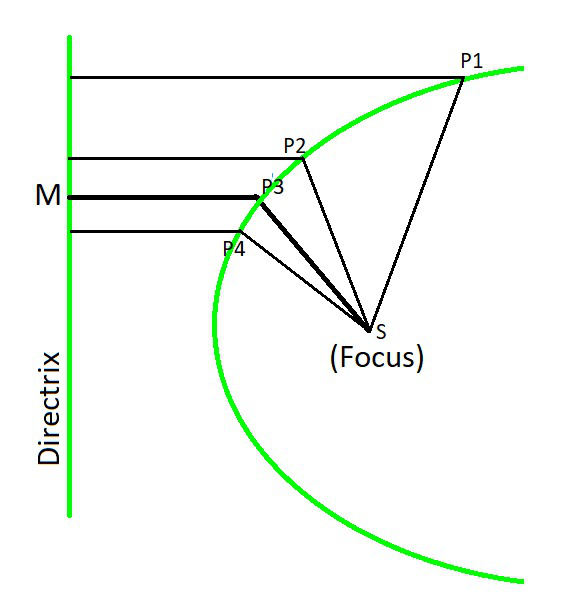### Equation of the parabola from focus & directrix

Now we will learn how to find the equation of the parabola from focus & directrix. So, let S be the focus, and the line ZZ’ be the directrix. Draw SK perpendicular from S on the directrix and bisect SK at V. Then,

VS = VK

The distance of V from the focus = Distance of V from the directrix

V lies on the parabola, So, SK = 2a.

Then, VS = VK = a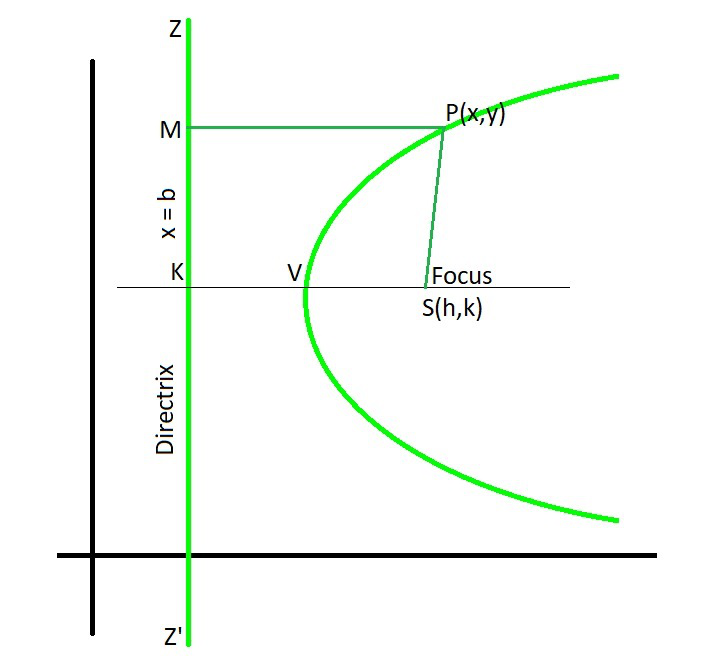Let’s take V as vertex, VK is a line perpendicular to ZZ’ and parallel to the x-axis. Then, the coordinates of focus S are (h, k) and the equation of the directrix ZZ’ is x = b. PM is perpendicular to directrix x = b and point M will be (b, y)

Let us considered a point P(x, y) on the parabola. Now, join SP and PM.

As we know that P lies on the parabola

So, SP = PM (Parabola definition)

SP2 = PM2

(x – h)2 + (y – k)2 = (x – b)2 + (y – y)2

x2 – 2hx + h2 + (y-k)2 = x2 – 2bx + b2

Add (2hx – b2) both side, we get

x2 – 2hx + h2 + 2hx – b2 + (y-k)2 = x2 – 2bx + b2 + 2hx – b2

2(h – b)x = (y-k)2 + h2 – b2

Divide equation by 2(h – b), we get

x =x =………………..(1)

Similarly when directrix y = b, we get

y =………………..(2)

When V is origin, VS as x-axis of length a. Then, the coordinates of S will be (a, 0), and directrix ZZ’ is x = -a.

h = a, k = 0 and b = -a

Using the equation (1), we get

x =x =y2 = 4ax

It is the standard equation of the parabola.

Note: The parabola has two real foci situated on its axis one of which is the focus S and the other lies at infinity. The corresponding directrix is also at infinity.

Tracing of the parabola y2 = 4ax, a>0

The given equation can be written as y = ± 2, we observe the following points from the equation:

• Symmetry: The given equation states that for every positive value of x, there are two equal and opposite value of y.
• Region: The given equation states that for every negative value of x, the value of y is imaginary which means no part of the curve lies to left of the y-axis.
• Origin: Origin is the point from where the curve passes through and the tangent at the origin is x = 0 i.e., y-axis.
• Portion occupied: As x⇢∞, y⇢∞. Hence the curve extends to infinity to the right of the axis of y.

### Some other standard forms of the parabola with focus and directrix

The simplest form of the parabola equation is when the vertex is at the origin and the axis of symmetry is along with the x-axis or y-axis. Such types of parabola are:

1. y2 = 4ax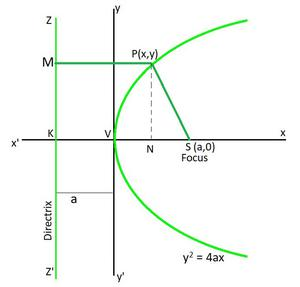Here,

• Coordinates of vertex: (0, 0)
• Coordinates of focus: (a, 0)
• Equation of the directrix: x = -a
• Equation of axis: y = 0
• Length of the latus rectum: 4a
• Focal distance of a point P(x, y): a + x

2. x2 = 4ay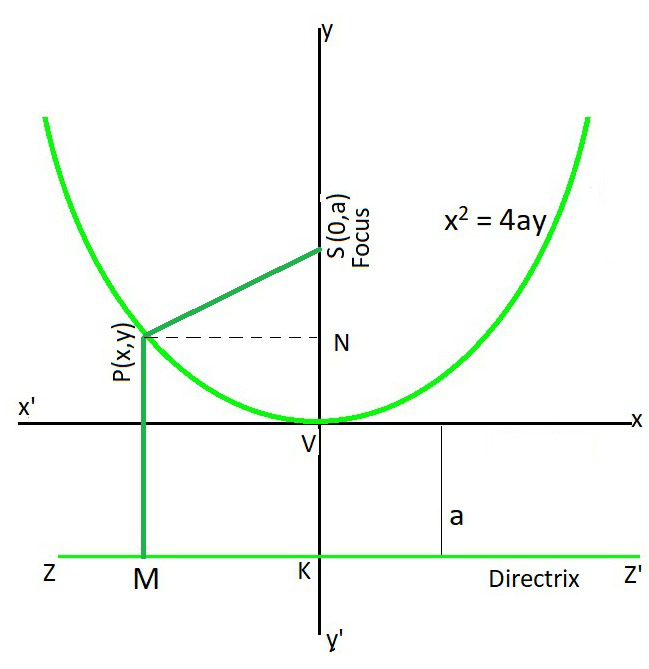Here,

• Coordinates of vertex: (0, 0)
• Coordinates of focus: (-a, 0)
• Equation of the directrix: x = a
• Equation of axis: y = 0
• Length of the latus rectum: 4a
• Focal distance of a point P(x, y): a – x

3. y2 = – 4ay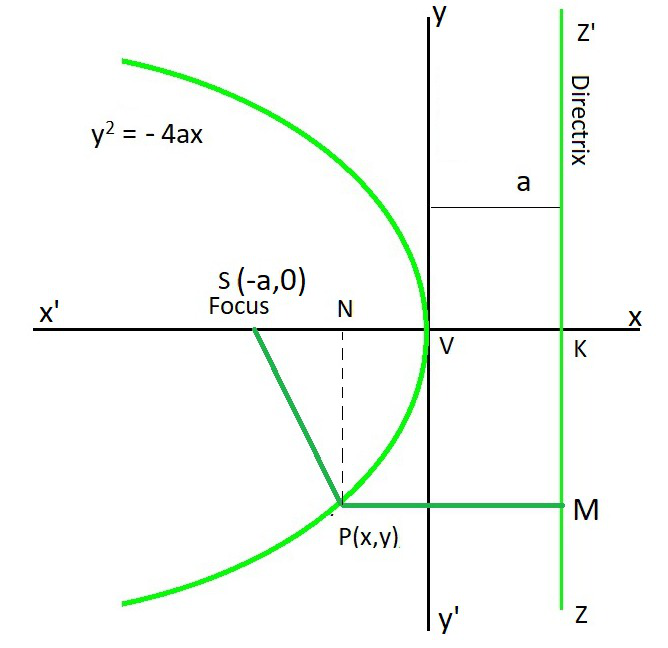Here,

• Coordinates of vertex: (0, 0)
• Coordinates of focus: (0, a)
• Equation of the directrix: y = -a
• Equation of axis: x = 0
• Length of the latus rectum: 4a
• Focal distance of a point P(x, y): a + y

4. x2 = – 4ay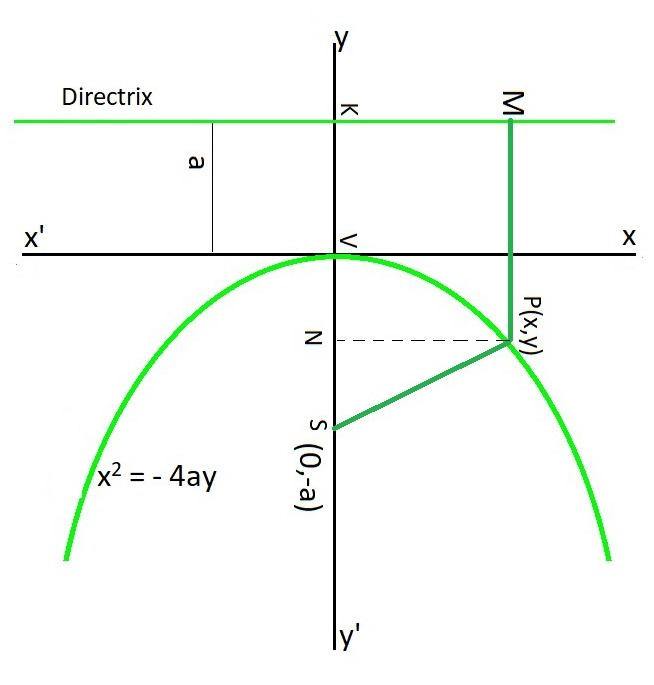Here,

• Coordinates of vertex: (0, 0)
• Coordinates of focus: (0, -a)
• Equation of the directrix: y = a
• Equation of axis: x = 0
• Length of the latus rectum: 4a
• Focal distance of a point P(x, y): a – y

### Sample Problems

Question 1. Find the equation of the parabola whose focus is (-4, 2) and the directrix is x + y = 3.

Solution:

Let P (x, y) be any point on the parabola whose focus is (-4, 2) and the directrix x + y – 3 = 0.

As we already know that the distance of a point P from focus = distance of a point P from directrix

So, √(x + 4)2 + (y – 2)2On squaring both side we get

(x + 4)2 + (y – 2)22((x2 + 16 + 8x) + (y2+ 4 – 4y)) = x2 + y2 + 9 +2xy – 6x – 6y

2(x2 + 20 + 8x + y2 – 4y) = x2 + y2 + 9 +2xy – 6x – 6y

2x2 + 40 + 16x + 2y2 – 8y = x2 + y2 + 9 +2xy – 6x – 6y

x2 + y2 + 2xy + 10x – 2y + 31 = 0

Question 2. Find the equation of the parabola whose focus is (-4, 0) and the directrix x + 6 = 0.

Solution:

Let P (x, y) be any point on the parabola whose focus is (-4, 0) and the directrix x + 6 = 0.

As we already know that the distance of a point P from focus = distance of a point P from directrix

So,  √(x + 4)2 + (y )2On squaring both side we get

(x + 4)2 + (y)22x2 + 32 + 16x + 2y2 = x2 + 36 + 12x

x2 + 2y2 – 4 + 14x = 0

Question 3. Find the equation of the parabola with focus (4, 0) and directrix x = – 3.

Solution:

Since the focus (4, 0) lies on the x-axis, the x-axis itself is the axis of the parabola.

Hence, the equation of the parabola is of the form either

y2 = 4ax or y2= – 4ax.

Since the directrix is x = – 3 and the focus is (4, 0),

the parabola is to be of the form y2= 4ax with a = 4.

Hence, the required equation is

y2 = 4(4)x

y2 = 16x

Question 4. Find the equation of the parabola with vertex at (0, 0) and focus at (0, 4).

Solution:

Since the vertex is at (0, 0) and the focus is at (0, 5) which lies on y-axis, the y-axis is the axis of the parabola.

Hence, the equation of the parabola is x2= 4ay.

Hence, we have x2 = 4(4)y, i.e.,

x2 = 16y

### Focus & directrix of a parabola from the equation

Now we will learn how to find the focus & directrix of a parabola from the equation.So, when the equation of a parabola is

y – k = a(x – h)2

Here, the value of a = 1/4C

So the focus is (h, k + C), the vertex is (h, k) and the directrix is y = k – C.

### Sample Examples

Question 1. y2 = 8x

Solution:

The given parabola is of the form y2 = 4ax, where

4a = 8

a = 2

The coordinates of the focus are (a,0),  i.e. (2,0)

and, the equation of the directrix is

x = -a, i.e. x = -2

Question 2. y2 – 8y – x + 19 = 0

Solution:

By rearranging, we get

y2 – 8y + 16 – x + 3 = 0

y2 – 8y + 16 = x – 3

x = (y-4)2 + 3

Comparing with eq(1), we conclude

k = 4

2(h-b) = 1 ……………(I)= 3 ……………(II)

Solving (I) and (II), we get

h =and b =Hence, Focus is (h,k) = (,4)

and, directrix x = b =Question 3. Find focus, directrix and vertex of the following equation: y = x2 – 2x + 3

Solution:

By rearranging, we get

y =x2 – 2x + 4 – 1

y =(x-1)2 + 2

Comparing with eq(4), we conclude

h = 1

y1 = 2

2(k-b) = 1 ……………(I)= 2 ……………(II)

Solving (I) and (II), we get

k =and b =Hence, Focus is (h,k) = ( 1,),

directrix y = b =and, vertex (h, y1) = (1,2)

Whether you're preparing for your first job interview or aiming to upskill in this ever-evolving tech landscape, GeeksforGeeks Courses are your key to success. We provide top-quality content at affordable prices, all geared towards accelerating your growth in a time-bound manner. Join the millions we've already empowered, and we're here to do the same for you. Don't miss out - check it out now!

Previous
Next